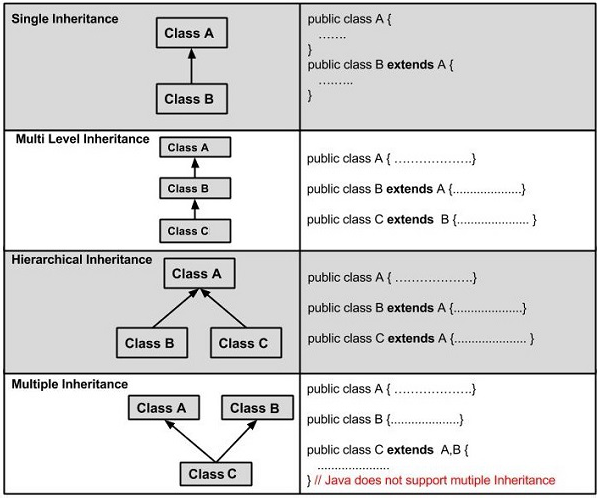# Java Tut – inheritance

The classs which inherits the properties of other is known as subclass(derived class,child class etc.) and the class whose properties are inherited is known as superclass(base class,parent class).

extends keyword:
extends is the keyword used to inherit the properties of a class, below given is the syntax of extends keyword:

```clas Super{

//code

}

class Sub extends Super{
//code

}```

example:
Calculation.java

```public class Calculation {
int z;
{
z=x+y;
System.out.println("The sum of the the given numers"+z);
}
public void subtraction(int x,int y)
{
z=x-y;
System.out.println("The difference between the given numbers:"+z);
}

}```

My_Calculation.java

```public class My_Calculation extends Calculation {

public void multiplication(int x,int y)
{
z=x*y;
System.out.println("The product of the given numbers:"+z);
}

public static void main(String[] args)
{
int a=20,b=10;
My_Calculation demo=new My_Calculation();
demo.subtraction(a, b);
demo.multiplication(a, b);
}

}
```

Output:

```The sum of the the given numers30
The difference between the given numbers:10
The product of the given numbers:200
```

Super keyword:
The super keyword is similar to this keyword, following are the scenarios where the super keyword is used

– it is ised to differentiate the members of superclass from the members of subclass, if they have same names,
– It is used to invoke the superclass contructor from subclass
Differentiating the members:
supe
super.method();

Super_classs.java

```public class Super_classs {
int num=20;
public void display()
{
System.out.println("This is the display method of superclass");
}
}
```

Sub_class.java

```public class Sub_class extends Super_classs {
int num=10;

public void display()
{
System.out.println("This is the display method of subclass");
}

public void myMethod()
{

Sub_class sub=new Sub_class(); //Instantiating subclass
sub.display(); //Invoking the display method of sub class
super.display();//invoking the display() method of superclass
System.out.println("value of the variable named num in sub class:"+sub.num);
System.out.println("value of the variable named num in the super class:"+super.num);

}
public static void main(String[] args)
{
Sub_class object_banaici=new Sub_class();
object_banaici.myMethod();
}

}
```

Output:

```This is the display method of subclass
This is the display method of superclass
value of the variable named num in sub class:10
value of the variable named num in the super class:20
```

Invoking Superclass constructor

If you want to call a parametrized constructor of the super class, you need to use the super keyword as shown below:
super(values);

Sample code:

SuperClass.java

```public class SuperClass {
int age;
SuperClass(int age)
{
this.age=age;
}

public void getAge(){
System.out.println("The value of the variable named age in super class is: "+age);
}

}
```

SubClass.java

```public class SubClass extends SuperClass{
SubClass(int age)
{
super(age);
}
public static void main(String[] args)
{
SubClass ss=new SubClass(24);
ss.getAge();
}
}
```

output:

```The value of the variable named age in super class is: 24
```

IS-A Relationship:
IS-A is a new way of saying: This object is a type of that object.Let us see how the extends keyword is used to achieve inheritance.

```public class Animal{
}

public class Mammal extends Animal{
}

public class Reptile extends Animal{
}

public class Dog extends Mammal{
}```

In object oriented terms, the following are true-
Animal is the superclass of Mammal class .
Animal is the superclass of Reptile class
Mammal and Reptile are subclass of Animal class
Dog is the subclass of both Mammal and ANimal classes
Now  if we consider the IS-A relationship,,,we can say-
-Mammal IS-A Animal
-Reptile IS-A Animal
-Dog IS-A Mammal
Hence: Dog IS-A Animal as well

Using the extends keyword the subclasses will inherit all the properties of super class except the private properties.

Now, we can ensure that Mammal is actually an Animal with the use of the instance operator.
Example:
Animal.java

```public class Animal {

}

class Mammal extends Animal{

}

class Reptile extends Animal{
}

```

Dogg.java

```public class Dogg extends Mammal{
public static void main(String[] args)
{
Animal a=new Animal();
Mammal m=new Mammal();
Dogg d=new Dogg();

System.out.println(m instanceof Animal);
System.out.println(d instanceof Mammal);
System.out.println(d instanceof Animal);
}

}```

Output:

```true
true
true
```

Since we have a good understanding of the extends keyword let us look into how the implements keyword is used to get the IS-A relationship.

Genrally the implements keyword s used with classes to inherit the properties of an inheritance.Interfaces can never be extended by a class.
Example:

```public interface Animal{
}

public class Mammal implements Animal{
}

public class Dog extends Mammal{
}
```

The instanceof keyword:

Let us use the instanceof operator to check determine whether Mammal is actually an Animal, and dog is actually an Animal

```interface Animals{}

class Mammal implements Animals{}

public class Dogg extends Mammal{
public static void main(String[] args)
{
Mammal m=new Mammal();
Dog d=new Dog();

System.out.println(m instanceof Animals);
System.out.println(d instanceof Mammal);
System.out.println(d instanceof Animals);

}
}```

Output:

```true
true
true```

Though there is some error in my above code.

HAS-A Relationship
These relationships are mainly based on the usage.This determines whether a certain class HAS-A certain thing.This relationship help to reduce duplication of codes as well as bugs.

let us look into an exampel:

```public class Vehicle{}
public class Speed{}

public class Vangari extends Vehicle{
private Speed sp;
}```

More details in the link below.
Types of inheritance:And one thing is Multiple Inheritance is not supported in java.It is illegal below:

`public class extends Animal,Mammal{}`

It would be a great help, if you support by sharing :)Author: zakilive